NEET  >  31 Year NEET Previous Year Questions: Nuclei - 1

# 31 Year NEET Previous Year Questions: Nuclei - 1

Test Description

## 30 Questions MCQ Test Physics Class 12 | 31 Year NEET Previous Year Questions: Nuclei - 1

31 Year NEET Previous Year Questions: Nuclei - 1 for NEET 2023 is part of Physics Class 12 preparation. The 31 Year NEET Previous Year Questions: Nuclei - 1 questions and answers have been prepared according to the NEET exam syllabus.The 31 Year NEET Previous Year Questions: Nuclei - 1 MCQs are made for NEET 2023 Exam. Find important definitions, questions, notes, meanings, examples, exercises, MCQs and online tests for 31 Year NEET Previous Year Questions: Nuclei - 1 below.
Solutions of 31 Year NEET Previous Year Questions: Nuclei - 1 questions in English are available as part of our Physics Class 12 for NEET & 31 Year NEET Previous Year Questions: Nuclei - 1 solutions in Hindi for Physics Class 12 course. Download more important topics, notes, lectures and mock test series for NEET Exam by signing up for free. Attempt 31 Year NEET Previous Year Questions: Nuclei - 1 | 30 questions in 60 minutes | Mock test for NEET preparation | Free important questions MCQ to study Physics Class 12 for NEET Exam | Download free PDF with solutions
 1 Crore+ students have signed up on EduRev. Have you?
31 Year NEET Previous Year Questions: Nuclei - 1 - Question 1

### In the reaction ,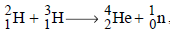if the binding energies of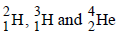are respectively, a, b and c (in MeV), then the energy (in MeV) released in this reaction is 

Detailed Solution for 31 Year NEET Previous Year Questions: Nuclei - 1 - Question 1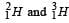requires a and b amount of energies for their nucleons to be separated.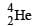releases c amount of energy in its formation i.e., in assembling the nucleons as nucleus.
Hence, Energy released =c – (a + b) = c – a – b

31 Year NEET Previous Year Questions: Nuclei - 1 - Question 2

### In any fission process, the ratio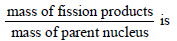Detailed Solution for 31 Year NEET Previous Year Questions: Nuclei - 1 - Question 2

Binding energy per nucleon for fission products is higher relative to Binding energy per nucleon for parent nucleus, i.e., more masses are lost and are obtained as kinetic energy of fission products. So, the given ratio < 1.

31 Year NEET Previous Year Questions: Nuclei - 1 - Question 3

### Fission of nuclei is possible because thebinding energy per nucleon in them 

Detailed Solution for 31 Year NEET Previous Year Questions: Nuclei - 1 - Question 3

Binding energy per nucleons decreases with increase in mass numbers.
The decrease of binding energy per nucleon for nuclei with a high mass number is due to increased Coulombic repulsion between protons inside the nucleus.

31 Year NEET Previous Year Questions: Nuclei - 1 - Question 4

The binding energy of deuteron is 2.2 MeV and that of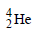is 28 MeV. If two deuterons are fused to form onethen the energy released is          

Detailed Solution for 31 Year NEET Previous Year Questions: Nuclei - 1 - Question 4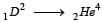Energy released = 28 – 2 × 2.2 = 23.6 MeV (Binding energy is energy released on formation of Nucleus)

31 Year NEET Previous Year Questions: Nuclei - 1 - Question 5

In a radioactive material the activity at time t1 is R1 and at a later time t2, it is R2. If the decay constant of the material is λ, then 

Detailed Solution for 31 Year NEET Previous Year Questions: Nuclei - 1 - Question 5

Let at time t1 & t2, number of particles be N1 & N2. So,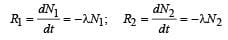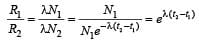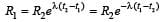31 Year NEET Previous Year Questions: Nuclei - 1 - Question 6

Two radioactive substances A and B have decay constants 5λ and λ respectively. At t = 0 they have the same number of nuclei. The ratio of number of nuclei of A to those of B will be (1/e)2 after a time interval 

Detailed Solution for 31 Year NEET Previous Year Questions: Nuclei - 1 - Question 6

λ A = 5λ and λB = λ
At t = 0,  (N0)A = (N0)B

Given,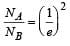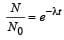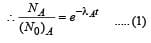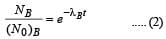From (1) and (2),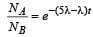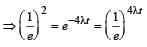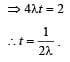31 Year NEET Previous Year Questions: Nuclei - 1 - Question 7

In a radioactive decay process, the negativelycharged emitted β -particles are 

Detailed Solution for 31 Year NEET Previous Year Questions: Nuclei - 1 - Question 7

In beta min us decay (β–), a neutron is transformed into a proton, and an electron is emitted from the nucleus along with antineutrino.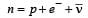31 Year NEET Previous Year Questions: Nuclei - 1 - Question 8

A nucleus AZX has mass represented by M (A, Z). If Mp and Mn denote the mass of proton and neutron respectively and B.E. the binding energy in MeV, then 

Detailed Solution for 31 Year NEET Previous Year Questions: Nuclei - 1 - Question 8

In the case of formation of a nucleus, the evolution of energy equal to the binding energy of the nucleus takes place due to the disappearance of a fraction of the total mass. If the quantity of mass disappearing is ΔM, then the binding energy is

BE = ΔMc2
From the above discussion, it is clear that the mass of the nucleus must be less than the sum of the masses of the constituent neutrons and protons. We can then write.

ΔM= [ZMp + (A-Z)Mn -M(A,Z)

Where M (A, Z)  is the mass of the atom of mass number A and atomic number Z. Hence, the binding energy of the nucleus is

BE = [ZMp + (A-Z)Mn -M(A,Z)]c2

Where N = A -Z = number of neutrons.

31 Year NEET Previous Year Questions: Nuclei - 1 - Question 9

If the nucleus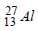has nuclear radius of about 3.6 fm, then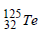would have its radius approximately as 

Detailed Solution for 31 Year NEET Previous Year Questions: Nuclei - 1 - Question 9

It has been known that a nucleus of mass number A has radius R = R0A1/3,
where R0 = 1.2 × 10–15m and A = mass number

In case of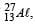let nuclear radius be R1

and for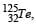nuclear radius be R2

ForFor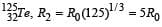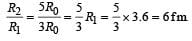31 Year NEET Previous Year Questions: Nuclei - 1 - Question 10

Two radioactive materials X1 and X2 have decay constants 5λ and λ respectively. If initially they have the same number of nuclei, then the ratio of the number of nuclei of X1 to that of X2 will be 1/e after a time

Detailed Solution for 31 Year NEET Previous Year Questions: Nuclei - 1 - Question 10

Let the required time be t. Then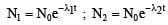Where N1 = number of nuclei of X1 after time t
N2 = number of nuclei of X2 after time t
N0 = initial number of nuclei of X1 and X2 each.

Now,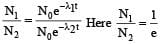λ1 = 5λ;    λ2 = λ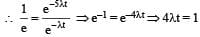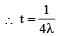31 Year NEET Previous Year Questions: Nuclei - 1 - Question 11

If M (A; Z), Mp and Mn denote the masses of the nucleus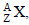proton and neutron respectively in units of u ( 1u = 931.5 MeV/c2) and BE represents its bonding energy in MeV, then 

Detailed Solution for 31 Year NEET Previous Year Questions: Nuclei - 1 - Question 11

Mass defect = ZMp + (A –Z)Mn–M(A,Z)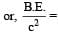ZMp + (A–Z) Mn–M(A,Z)

∴ M (A, Z) = ZMp + (A–Z)Mn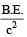31 Year NEET Previous Year Questions: Nuclei - 1 - Question 12

Two nuclei have their mass numbers in the ratioof 1 : 3. The ratio of their nuclear densities wouldbe 

Detailed Solution for 31 Year NEET Previous Year Questions: Nuclei - 1 - Question 12

Requird ratio of nuclear densities =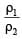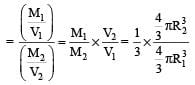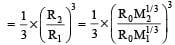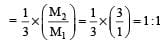31 Year NEET Previous Year Questions: Nuclei - 1 - Question 13

In the nuclear decay given below: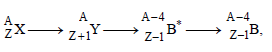the particles emitted in the sequence are

Detailed Solution for 31 Year NEET Previous Year Questions: Nuclei - 1 - Question 13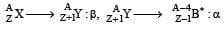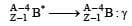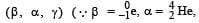mass number and charge number of a nucleus remains unchanged during γ decay)

31 Year NEET Previous Year Questions: Nuclei - 1 - Question 14

The mass of a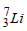nucleus is 0.042 u less than the sum of the masses of all its nucleons. The binding energy per nucleon ofnucleus is nearly

Detailed Solution for 31 Year NEET Previous Year Questions: Nuclei - 1 - Question 14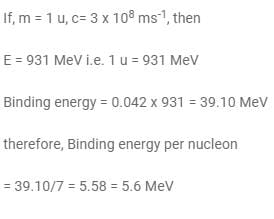31 Year NEET Previous Year Questions: Nuclei - 1 - Question 15

The activity of a radioactive sample is measured as N0 counts per minute at t = 0 and N0/e counts per minute at t = 5 minutes. The time (in minutes) at which the activity reduces to half its value is   

Detailed Solution for 31 Year NEET Previous Year Questions: Nuclei - 1 - Question 15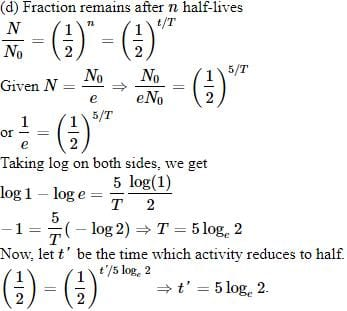31 Year NEET Previous Year Questions: Nuclei - 1 - Question 16

The binding energy per nucleon in deuteriumand helium nuclei are 1.1 MeV and 7.0 MeV,respectively. When two deuterium nuclei fuseto form a helium nucleus the energy released inthe fusion is :

Detailed Solution for 31 Year NEET Previous Year Questions: Nuclei - 1 - Question 16

Binding energy of two 1H2 nuclei = 2(1.1 × 2) = 4.4 MeV
Binding energy of one 2He4 nucelus = 4 × 7.0 =28 MeV
∴ Energy released = 28 – 4.4 = 23.6 MeV

31 Year NEET Previous Year Questions: Nuclei - 1 - Question 17

The decay constant of a radio isotope is λ. If A1 and A2 are its activities at times t1 and t2 respectively, the number of nuclei which have decayed during the time (t1 – t2) :

Detailed Solution for 31 Year NEET Previous Year Questions: Nuclei - 1 - Question 17

Activity is given by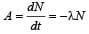Activity at time t1 is A1 =- λN1
and activity at time t2 is A2 = – l N2 As t1 > t2, therefore, number of atoms remained after time t1 is less than that remained after time t2. That is, N1 < N2.
∴ number of nuclei decayed in (t1 – t2)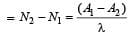31 Year NEET Previous Year Questions: Nuclei - 1 - Question 18

The half life of a radioactive isotope 'X' is 50 years. It decays to another element 'Y' which isstable. The two elements 'X' and 'Y' were foundto be in the ratio of 1 : 15 in a sample of agiven rock. The age of the rock was estimatedto be 

Detailed Solution for 31 Year NEET Previous Year Questions: Nuclei - 1 - Question 18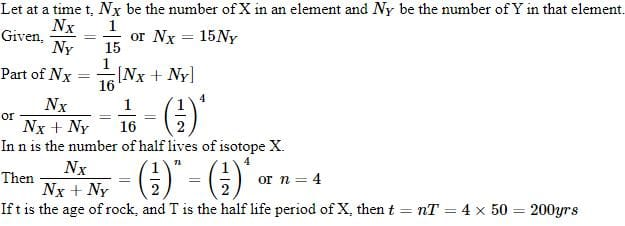31 Year NEET Previous Year Questions: Nuclei - 1 - Question 19

The power obtained in a reactor using U235 disintegration is 1000 kW. The mass decay of U235 per hour is 

Detailed Solution for 31 Year NEET Previous Year Questions: Nuclei - 1 - Question 19

E = mc2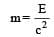So, mass decay per second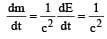(Power in watt)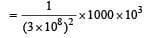and mass decay per hour =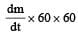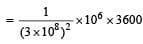= 4 × 10–8 kg

= 40 microgram

31 Year NEET Previous Year Questions: Nuclei - 1 - Question 20

A radioactive nucleus of mass M emits a photonof frequency μ and the nucleus recoils. The recoilenergy will be 

Detailed Solution for 31 Year NEET Previous Year Questions: Nuclei - 1 - Question 20

Momentum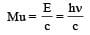Recoil energy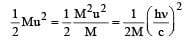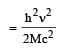31 Year NEET Previous Year Questions: Nuclei - 1 - Question 21

A nucleus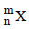emits one α-particle and two β-particles. The resulting nucleus is 

Detailed Solution for 31 Year NEET Previous Year Questions: Nuclei - 1 - Question 21

When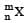emits one -particle then its atomic mass decreases by 4 units and atomic number by 2. Therefore, the new nucleus becomes mn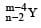. But as it emits two β particles, its atomic number increases by 2. Thus the resulting nucleus is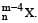31 Year NEET Previous Year Questions: Nuclei - 1 - Question 22

Fusion reaction takes place at high temperaturebecause    

Detailed Solution for 31 Year NEET Previous Year Questions: Nuclei - 1 - Question 22

When the coulomb repulsion between the nuclei is overcome then nuclear fusion reaction takes place. This is possible when temperature is too high.

31 Year NEET Previous Year Questions: Nuclei - 1 - Question 23

Two radioactive nuclei P and Q, in a given sample decay into a stable nucleolus R. At time t = 0, number of P species are 4 N0 and that of Q are N0. Half-life of P (for conversion to R) is 1 minute where as that of Q is 2 minutes. Initially there are no nuclei of R present in the sample. When number of nuclei of P and Q are equal, the number of nuclei of R present in the sample would be [2011M]

Detailed Solution for 31 Year NEET Previous Year Questions: Nuclei - 1 - Question 23

Initially  P → 4N0
Q → N0
Half life TP = 1 min.
TQ = 2 min.
Let after time t number of nuclei of P and Q are equal, that is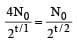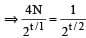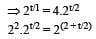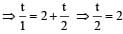⇒ t = 4 min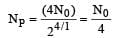at t = 4 min.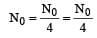or population of R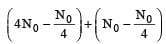31 Year NEET Previous Year Questions: Nuclei - 1 - Question 24

If the nuclear radius of 27Al is 3.6 Fermi, the approximate nuclear radius of 64Cu in Fermi is :      

Detailed Solution for 31 Year NEET Previous Year Questions: Nuclei - 1 - Question 24

The radius of the nuclears is directly proportional to cube root of  atomic number i.e. R ∝ A1/3 ⇒ R = R0 A1/3, where R0 is a constant of proportionality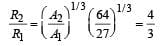where R1 = the radius of 27Al, and A1 = Atomic  mass number of Al R2 = the radius of 64Cu and A2 = Atomic mass number of C4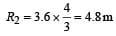31 Year NEET Previous Year Questions: Nuclei - 1 - Question 25

A mixture consists of two radioactive materials A1 and A2 with half lives of 20 s and 10 srespectively. Initially the mixture has 40 g of Aand 160 g of A2. The amount of the two in themixture will become equal after : 

Detailed Solution for 31 Year NEET Previous Year Questions: Nuclei - 1 - Question 25

Let, the amount of the two in the mixture will become equal after t years.
The amount of A1, which remains after t years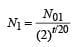The amount of A2, which remains, after t years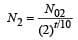According to the problem N1 = N2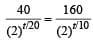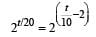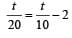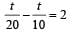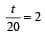t = 40 s

31 Year NEET Previous Year Questions: Nuclei - 1 - Question 26

The half life of a radioactive nucleus is 50 days.  The time interval (t2 – t1) between the time twhen 2/3 of its has decayed and the time t1 when 1/3  of it had decayed is :      

Detailed Solution for 31 Year NEET Previous Year Questions: Nuclei - 1 - Question 26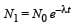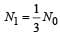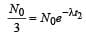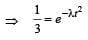...(i)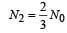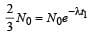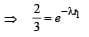...(ii)

Dividing equation (i) by equation (ii)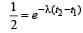λ (t2 - t1) = ln2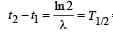= 50 days

31 Year NEET Previous Year Questions: Nuclei - 1 - Question 27

A certain mass of Hydrogen is changed to Heliumby the process of fusion. The mass defect infusion reaction is 0.02866 a.m.u. The energyliberated per a.m.u. is(Given : 1 a.m.u = 931 MeV)  [NEET 2013]

Detailed Solution for 31 Year NEET Previous Year Questions: Nuclei - 1 - Question 27

Mass defect Δm = 0.02866 a.m.u.
Energy = 0.02866 × 931 = 26.7 MeV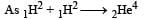Energy liberated per a.m.u = 13.35/2 MeV
= 6.675 MeV

31 Year NEET Previous Year Questions: Nuclei - 1 - Question 28

The half life of a radioactive isotope ‘X’ is20 years. It decays to another element ‘Y’ whichis stable. The two elements ‘X’ and ‘Y’ were foundto be in the ratio of 1 : 7 in a sample of a thegiven rock. The age of the rock is estimated tobe [NEET 2013]

Detailed Solution for 31 Year NEET Previous Year Questions: Nuclei - 1 - Question 28

The value of x is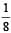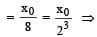t = 3T = 3 × 20 = 60
years Hence the estimated age of the rock is 60 years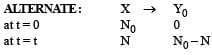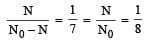t = 3T = 3 × 20 = 60 years

31 Year NEET Previous Year Questions: Nuclei - 1 - Question 29

α-particles, β-particles and γ-rays are all havingsame energy. Their penetrating power in a givenmedium in increasing order will be   [NEET Kar. 2013]

Detailed Solution for 31 Year NEET Previous Year Questions: Nuclei - 1 - Question 29

Increasing order of penetrating power : α < β < γ.
For same energy, lighter particle has higher penentrating power.

31 Year NEET Previous Year Questions: Nuclei - 1 - Question 30

How does the binding energy per nucleon varywith the increase in the number of nucleons  ?   [NEET Kar. 2013]

Detailed Solution for 31 Year NEET Previous Year Questions: Nuclei - 1 - Question 30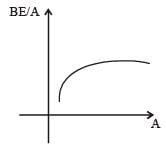From the graph of BE/A versus mass number A  it is clear that, BE/A first increases and then decreases with increase in mass number.

## Physics Class 12

157 videos|452 docs|213 tests
 Use Code STAYHOME200 and get INR 200 additional OFF Use Coupon Code
Information about 31 Year NEET Previous Year Questions: Nuclei - 1 Page
In this test you can find the Exam questions for 31 Year NEET Previous Year Questions: Nuclei - 1 solved & explained in the simplest way possible. Besides giving Questions and answers for 31 Year NEET Previous Year Questions: Nuclei - 1, EduRev gives you an ample number of Online tests for practice

## Physics Class 12

157 videos|452 docs|213 tests

### How to Prepare for NEET

Read our guide to prepare for NEET which is created by Toppers & the best Teachers